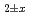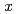# Oxygen potentials, oxygen diffusion coefficients and defect equilibria of nonstoichiometric (U,Pu)OOxygen potential of (U,Pu)Owas evaluated based on defect chemistry using an updated experimental data set. The relationship between oxygen partial pressure and deviationin (U,Pu)Owas analyzed, and equilibrium constants of defect formation were determined as functions of Pu content and temperature. Brouwer's diagrams were constructed using the determined equilibrium constants, and a relational equation to determine O/M ratio was derived as functions of O/M ratio, Pu content and temperature. In addition, relationship between oxygen potential and oxygen diffusion coefficients were described.